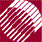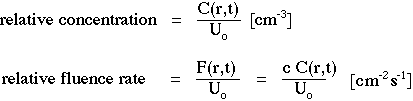ECE532 Biomedical Optics © 1998 Steven L. Jacques, Scott A. Prahl Oregon Graduate Institute

# Time-resolved Monte Carlo

## Relative concentration and fluence rate

In time-resolved Monte Carlo simulations, we record the time-resolved spatial distribution of photons expressed as either an energy concentration (C [J/cm3]) or an instantaneous fluence rate (F [W/cm2]) relative to the impulse energy (Uo [J]) of the source at time zero:If one wishes to do bookkeeping of C as [photons cm-3] or F as [photons cm-2 s-1], then one simply multiplies the Monte Carlo result, C/Uo or F/Uo, by the value of one's source expressed as number of photons, for example, a 1-J impulse at 532 nm wavelength:

Uo = (1 J) / ( hc/λ )

= (1 J) / ( (6.626x10-34 Js)(2.998x108 m/s) / (532x10-9 m) )

= 2.7x1018 photons.

NextMonte Carlo